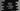# How to check if a triangle is valid or not in python## How to check if a triangle is valid or not in python :

In this tutorial, we will learn how to check if a triangle is valid or not in Python. The program will take the angles as input from the user and print out one message if it is valid or not.

The basic idea is to check the sum of all angles that we received is equal to 180 or not. If yes, then this is a valid, else it is not.

Also, we have to check if all angles are greater than 0 or not. Because, we can’t have a triangle with 0 degree angle.

So the algorithm of this program is :

• Take the angles of the triangle one by one.
• Using a if-else check, find if the sum of the angles is 180 or not. Also, check if all angles are greater than 0 or not.
• Based on that if-else block, print one message if it is a valid triangle or not.

### Python program:

Below is the complete python program:

``````first_angle = float(input("Enter the first angle : "))
second_angle = float(input("Enter the second angle : "))
third_angle = float(input("Enter the third angle : "))

if first_angle + second_angle + third_angle == 180 and first_angle != 0 and second_angle != 0 and third_angle != 0:
print("Angles are valid for a triangle")
else:
print("Invalid angles")``````
• we are reading the user input as float.
• firstangle, secondangle and third_angle variables are used to store the angles for the triangle by taking the value as user input.
• The if condition checks if the sum of these angles are 180 and all are not equal to 0 or not. If yes, then we are printing that the angles are valid . Else, we are printing that they are invalid.

### Sample Output:

``````Enter the first angle : 179.5
Enter the second angle : .4
Enter the third angle : .1
Angles are valid for a triangle

Enter the first angle : 90
Enter the second angle : 90
Enter the third angle : 0
Invalid angles

Enter the first angle : 90
Enter the second angle : 60
Enter the third angle : 30
Angles are valid for a triangle``````